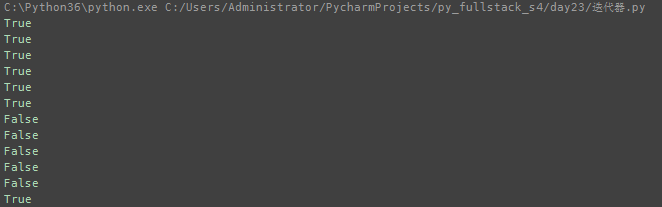Python学习之三大名器-装饰器、迭代器、生成器

import time
#定义装饰器
def timer(func):
def wrapper(*args,**kwargs):
start_time = time.time()
res = func(*args,**kwargs)
stop_time = time.time()
print("run time is %s" %(stop_time-start_time))
return res
return wrapper
#调用装饰器
@timer
def index():
l = []
for i in range(10000000):
l.append(i)
#调用阶段
index()

def auth2(auth_type): #1 #3
def auth(func): #4 #6
def wrapper(*args,**kwargs): #7 #10
if auth_type == 'file': #11
if name == 'zhejiangF4' and password == '666':
print('auth successfull')
res=func(*args,**kwargs)
return res
else:
print('auth error')
elif auth_type == 'sql': #12
print('nothing！') #13
return wrapper #8
return auth #5
@auth2(auth_type='sql') #2
def index():
print('welcome to inex page')
index() #9

1：迭代器提供了一种不依赖于索引的取值方式，这样就可以遍历那些没有索 引的可迭代对象了（字典，集合，文件）
2：迭代器与列表比较，迭代器是惰性计算的，更节省内存

**缺点：
1：无法获取迭代器的长度，使用不如列表索引取值灵活
2**：**一次性的，只能往后取值，不能倒着取值**

from collections import Iterable,Iterator
s='hello'
l=[1,2,3]
t=(1,2,3)
d={'a':1}
set1={1,2,3,4}
f=open('a.txt')
s.__iter__()
l.__iter__()
t.__iter__()
d.__iter__()
set1.__iter__()
f.__iter__()
print(isinstance(s,Iterable))
print(isinstance(l,Iterable))
print(isinstance(t,Iterable))
print(isinstance(d,Iterable))
print(isinstance(set1,Iterable))
print(isinstance(f,Iterable))
print(isinstance(s,Iterator))
print(isinstance(l,Iterator))
print(isinstance(t,Iterator))
print(isinstance(d,Iterator))
print(isinstance(set1,Iterator))
print(isinstance(f,Iterator))yield与return有何区别？
return只能返回一次函数就彻底结束了，而yield能返回多次值
yield到底干了什么事情：
yield把函数变成生成器（生成器就是迭代器）

from urllib.request import urlopen
def get(url):
while True:
def index():
url = yield index()
g = get('http://www.baidu.com')
next(g)
def run():
while True:
url = input("请输入URL：")
if 'http://' not in url:
print(g.send('http://'+url))
else:
print(g.send(url))
run()

def cat(filename):
with open(filename,'r') as f:
while True:
if not line:
break
else:
yield line
def grep(string,lines):
for line in lines:
if string in line:
yield line
g1 = cat('a.txt')
g2 = grep('mac',g1)
if __name__ == '__main__':
m = input("请输入命令：").strip()
if m == "cat a.txt |grep mac":
for i in g2:
print(i)

def hello(func):
def wrapper(*args,**kwargs):
res = func(*args,**kwargs)
next(res)
return res
return wrapper
@hello
def eater(name):
print('%s start to eat food' %name)
food_list=[]
while True:
food=yield food_list
print('%s get %s ,to start eat' %(name,food))
food_list.append(food)
print('done')
e=eater("somebody")
print(e.send('巧克力'))
print(e.send("香蕉"))

• 博文量
167
• 访问量
101945